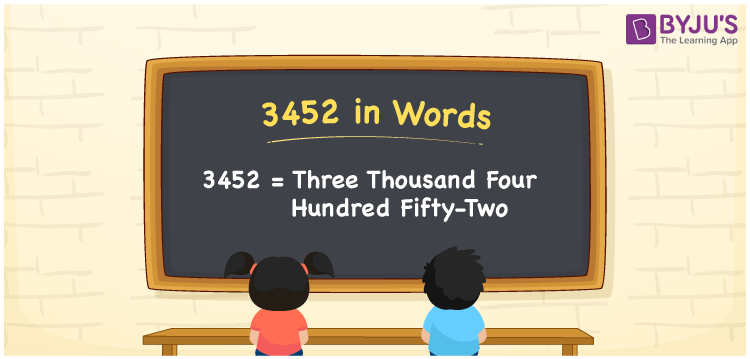# 3452 in Words

We can write 3452 in words as Three thousand four hundred fifty-two. If you have submitted a report that contains 3452 words, then you can say, “I submitted a report of Three thousand four hundred fifty-two words”. We can say that the word form of 3452 is used to express or communicate that number. In this article, you will get the conversion of the cardinal number 3452 into words with the help of a place value chart.

 3452 in words Three thousand four hundred fifty-two Three thousand four hundred fifty-two in Numbers 3452

## 3452 in English Words

We generally express numbers in words using the English alphabet. So, we can write 3452 in English words as “Three thousand four hundred fifty-two”.## How to Write 3452 in Words?

The below table shows the place values for all the four digits of the number 3452. Using this table, we can derive the number 3452 to word form.

 Thousands Hundreds Tens Ones 3 4 5 2

Here, ones = 2, tens = 5, hundreds = 4, thousands = 3

These digits can be expanded as:

3 × Thousand + 4 × Hundred + 5 × Ten + 2 × One

= 3 × 1000 + 4 × 100 + 5 × 10 + 2 × 1

= 3000 + 400 + 50 + 2

= Three thousand + Four hundred + Fifty + Two

= Three thousand four hundred fifty-two

Therefore, 3452 in words = Three thousand four hundred fifty-two.

As we know, 3452 is a natural number that precedes 3453 and succeeds 3451.

3452 in words – Three thousand four hundred fifty-two

Is 3452 an odd number? – No

Is 3452 an even number? – Yes

Is 3452 a perfect square number? – No

Is 3452 a perfect cube number? – No

Is 3452 a prime number? – No

Is 3452 a composite number? – Yes

## Frequently Asked Questions on 3452 in Words

Q1

### How do you spell 3452?

You can spell the number 3452 in English words as Three thousand four hundred fifty-two.
Q2

### How to write an amount Rs. 3452 in words on a cheque?

On a cheque, we generally write an amount Rs. 3452 in words as “Three thousand four hundred fifty-two rupees”.
Q3

### What is 3452 in word form?

Three thousand four hundred fifty-two represents the number 3452 in word form.